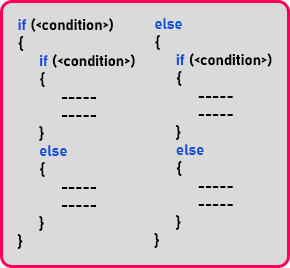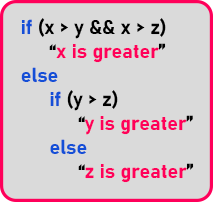# Nested If in C++

## Nested If Conditional Statement in C++ with Examples

In this article, I am going to discuss Nested If Conditional Statement in C++ with Examples. Please read our previous article where we discussed Compound Conditional Statement in C++ with Examples.

##### Nested if Conditional Statement:This is nested if conditional statement. As you can see, inside if, again there is an ’if-else block’. Also, inside the else part, again we have written ’if-else block’. So, we can write the conditions under the condition block. It depends on our problem or our requirement.

##### Can we write ‘if’ again inside nested if?

Yes, we can write ‘if – else’ up to any nested level. This is supported by C++. In some cases, we need to write nested conditional statements like this. And most of the time it happens to be inside the ‘else’ block rather than the ‘if’ block. We will be using this type of conditional statement many times in our program. Now let us take an example and use nested if statements for writing a program.

Let us write the program for finding ‘Greatest of 3 Numbers’. Let us have 3 numbers as input i.e. ‘x’, ‘y’, and ‘z’. We have these 3 variables and we want to find in which variable we have the greatest number. Let assign some values in these variables. ‘x = 10’, ‘y = 5’ and ‘z = 3’. Here ‘x’ has the greatest value among all three variables.

##### Pseudocode for Finding Greatest of 3 Numbers Problem:So here we simply defined our logic as, if ‘x’ is greater than both ‘y’ and ‘z’ then we will print ‘x is greater’ otherwise we will check for ‘y’ and ‘z’ in the else part as shown in above pseudo-code. Now before writing the program let us first understand the flowchart.

##### Flowchart for Finding Greatest of 3 Numbers:So, this is all we can check the greatest of three numbers. In this flowchart, first, we take input from the user by printing the message “Enter 3 no.” and store them in x, y, and z variables. Next, we check for if (x > y && y > z), if this condition is true then it will print the value of ‘x’ otherwise it will check for another condition that is if (y > z), if yes then it will print the value of ‘y’ and if it fails then it will print the value of ‘z’. So, there are two conditional statements. So let us see how to write this in a C++ program.

##### Finding Greatest of 3 Numbers Program Code:
```#include <iostream>
using namespace std;
int main()
{
int x, y, z;

cout << "Enter 3 no.s" << endl;
cin >> x >> y >> z;

if (x > y && x > z)
{
cout << x << endl;
}
else if (y > z)
{
cout << y << endl;
}
else
{
cout << z << endl;
}

return 0;
}
```
###### Output:In the next article, I am going to discuss How to Find the Nature of Quadratic Roots in C++ with Examples. Here, in this article, I try to explain Nested If Conditional Statement in C++ with Examples and I hope you enjoy this Nested If Conditional Statement in C++ with Examples article.